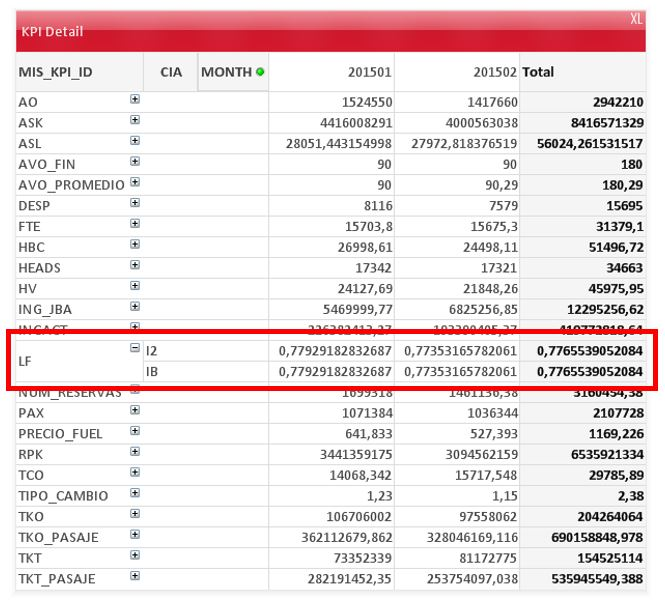# QlikView Creating Analytics

Discussion Board for collaboration related to Creating Analytics for QlikView.Not applicable

## Aggregate function

Hi all!

I have a pivot table with a conditional expresion, one of the calculations is an Aggr function, the expression works, but when i'm going to do drilldown for a different dimension, the value of the dimensions are the same, only shows the total value.

How can i create this expression?, i need to do drilldown.

This is the expression:

=if(MIS_KPI_ID='LF',

(

Sum(TOTAL <MONTH> Aggr(Sum(TOTAL <MONTH,MIS_KPI_ID,CIA_KPI> {<MIS_KPI_ID = {'RPK'}>} VALUE),MIS_KPI_ID,MONTH,CIA_KPI))

/

Sum(TOTAL <MONTH> Aggr(Sum(TOTAL <MONTH,MIS_KPI_ID,CIA_KPI> {<MIS_KPI_ID = {'ASK'}>} VALUE),MIS_KPI_ID,MONTH,CIA_KPI))

),Sum(VALUE)

)

...and this the result of the pivot table:Thanks for the help.

Best Regards.

1 Solution

Accepted SolutionsMVP

## Re: Aggregate function

Use this expression (PFA)

=if(MIS_KPI_ID='LF',

(

Sum(TOTAL <MONTH, CIA_KPI> Aggr(Sum({<MIS_KPI_ID = {'RPK'}>} VALUE),MONTH, CIA_KPI, MIS_KPI_ID)) /

Sum(TOTAL <MONTH, CIA_KPI> Aggr(Sum({<MIS_KPI_ID = {'ASK'}>} VALUE),MONTH, CIA_KPI, MIS_KPI_ID))

)

,

Sum(VALUE)

)

6 RepliesMVP

## Re: Aggregate function

I think you need to play around with the second total within Aggr(Sum( Total....)) to get it to give you the proper result.

Try removing and adding them one by one and see if that makes any difference:

=if(MIS_KPI_ID='LF',

(

Sum(TOTAL <MONTH> Aggr(Sum(TOTAL <MONTH,MIS_KPI_ID,CIA_KPI> {<MIS_KPI_ID = {'RPK'}>} VALUE),MIS_KPI_ID,MONTH,CIA_KPI))

/

Sum(TOTAL <MONTH> Aggr(Sum(TOTAL <MONTH,MIS_KPI_ID,CIA_KPI> {<MIS_KPI_ID = {'ASK'}>} VALUE),MIS_KPI_ID,MONTH,CIA_KPI))

),Sum(VALUE)

)

We might be able to give you a better solution if you can share a sample app.

Best,

SunnyNot applicable

## Re: Aggregate function

I cannot make it work, see attachment.

Bests Regards.MVP

## Re: Aggregate function

Use this expression (PFA)

=if(MIS_KPI_ID='LF',

(

Sum(TOTAL <MONTH, CIA_KPI> Aggr(Sum({<MIS_KPI_ID = {'RPK'}>} VALUE),MONTH, CIA_KPI, MIS_KPI_ID)) /

Sum(TOTAL <MONTH, CIA_KPI> Aggr(Sum({<MIS_KPI_ID = {'ASK'}>} VALUE),MONTH, CIA_KPI, MIS_KPI_ID))

)

,

Sum(VALUE)

)Not applicable

## Re: Aggregate function

Thanks for your workaround, the solution works but has a strange behavior when you're going to do drilldown on the kpi calculated. If you're going to do drilldown in LF... disappears.

If you click on "show all"... reappears.

What could be happening?

Bests Regards.MVP

## Re: Aggregate function

It is strange. I have never seen this kind of behavior like this before and not sure what is causing this. I might have to play around with it to see the potential cause of this. Maybe you can create another pivot chart and see if the behavior is repeated or not.

Best,

SunnyNot applicable

## Re: Aggregate function

Thanks sunindia‌, the cause is because it's marked the option to delete nulls.